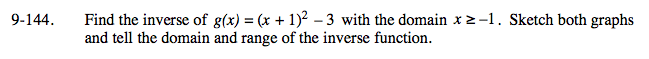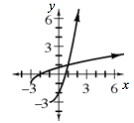### Home > A2C > Chapter 9 > Lesson 9.3.2 > Problem9-144

9-144.

Find the inverse of g(x) = (x + 1)2 − 3 with the domain x ≥ −1. Sketch both graphs and tell the domain and range of the inverse function. Homework Help ✎First switch the placement of x and y.

x = (y + 1)2 − 3

x + 3 = (y + 1)2

$y+1=\sqrt{x+3}$$\textit{y}=\sqrt{\textit{x}+3}-1$

D: x ≥ −3,
R: y ≥ −1